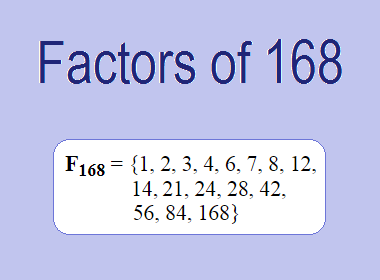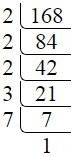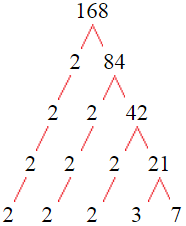# Factors of 168The factors of 168 are 1, 2, 3, 4, 6, 7, 8, 12, 14, 21, 24, 28, 42, 56, 84, and 168 i.e. F168 = {1, 2, 3, 4, 6, 7, 8, 12, 14, 21, 24, 28, 42, 56, 84, 168}. The factors of 168 are all the numbers that can divide 168 without leaving a remainder.

We can check if these numbers are factors of 168 by dividing 168 by each of them. If the result is a whole number, then the number is a factor of 168. Let's do this for each of the numbers listed above:

·        1 is a factor of 168 because 168 divided by 1 is 168.

·        2 is a factor of 168 because 168 divided by 2 is 84.

·        3 is a factor of 168 because 168 divided by 3 is 56.

·        4 is a factor of 168 because 168 divided by 4 is 42.

·        6 is a factor of 168 because 168 divided by 6 is 28.

·        7 is a factor of 168 because 168 divided by 7 is 24.

·        8 is a factor of 168 because 168 divided by 8 is 21.

·        12 is a factor of 168 because 168 divided by 12 is 14.

·        14 is a factor of 168 because 168 divided by 14 is 12.

·        21 is a factor of 168 because 168 divided by 21 is 8.

·        24 is a factor of 168 because 168 divided by 24 is 7.

·        28 is a factor of 168 because 168 divided by 28 is 6.

·        42 is a factor of 168 because 168 divided by 42 is 4.

·        56 is a factor of 168 because 168 divided by 56 is 3.

·        84 is a factor of 168 because 168 divided by 84 is 2.

·        168 is a factor of 168 because 168 divided by 168 is 1.

## How to Find Factors of 168?

1 and the number itself are the factors of every number. So, 1 and 168 are two factors of 168. To find the other factors of 168, we can start by dividing 168 by the numbers between 1 and 168. If we divide 168 by 2, we get a remainder of 0. Therefore, 2 is a factor of 168. If we divide 168 by 3, we get a remainder of 0. Therefore, 3 is also a factor of 168.

Next, we can check if 4 is a factor of 168. If we divide 168 by 4, we get a remainder of 0. Therefore, 4 is also a factor of 168. If we divide 168 by 5, we get a remainder of 3. Therefore, 5 is not a factor of 168. We can continue this process for all the possible factors of 168.

Through this process, we can find that the factors of 168 are 1, 2, 3, 4, 6, 7, 8, 12, 14, 21, 24, 28, 42, 56, 84, and 168. These are the only numbers that can divide 168 without leaving a remainder.

********************

********************

## Properties of the Factors of 168

The factors of 168 have some interesting properties. One of the properties is that the sum of the factors of 168 is equal to 480. We can see this by adding all the factors of 168 together:

1 + 2 + 3 + 4 + 6 + 7 + 8 + 12 + 14 + 21 + 24 + 28 + 42 + 56 + 84 + 168 = 480

Another property of the factors of 168 is that the prime factors of 168 are 2, 3, and 7 only.

## Applications of the Factors of 168

The factors of 168 have several applications in mathematics. One of the applications is in finding the highest common factor (HCF) of two or more numbers. The HCF is the largest factor that two or more numbers have in common. For example, to find the HCF of 168 and 84, we need to find the factors of both numbers and identify the largest factor they have in common. The factors of 168 are 1, 2, 3, 4, 6, 7, 8, 12, 14, 21, 24, 28, 42, 56, 84, and 168. The factors of 84 are 1, 2, 3, 4, 6, 7, 12, 14, 21, 28, 42, and 84. The largest factor that they have in common is 84. Therefore, the HCF of 168 and 84 is 84.

Another application of the factors of 168 is in prime factorization. Prime factorization is the process of expressing a number as the product of its prime factors. The prime factors of 168 are 2, 3, and 7, since these are the only prime numbers that can divide 168 without leaving a remainder. Therefore, we can express 168 as:

168 = 2 × 2 × 2 × 3 × 7

We can do prime factorization by division and factor tree method also. Here is the prime factorization of 168 by division method,168 = 2 × 2 × 2 × 3 × 7

Here is the prime factorization of 168 by the factor tree method,168 = 2 × 2 × 2 × 3 × 7

## Conclusion

The factors of 168 are the numbers that can divide 168 without leaving a remainder. The factors of 168 are 1, 2, 3, 4, 6, 7, 8, 12, 14, 21, 24, 28, 42, 56, 84, and 168. The factors of 168 have some interesting properties, such as having a sum of 480. The factors of 168 have several applications in mathematics, such as finding the highest common factor and prime factorization.